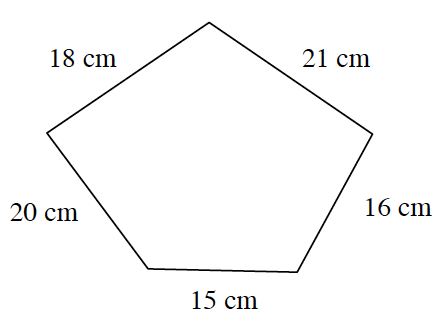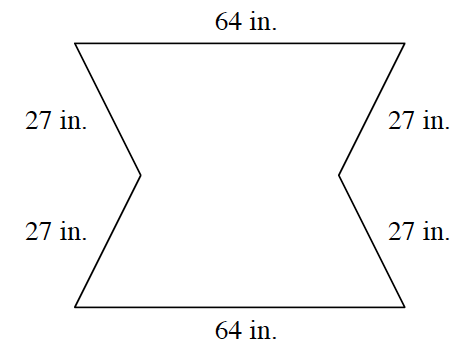### Home > MC1 > Chapter Ch2 > Lesson 2.1.4 > Problem2-47

2-47.

Find the perimeter (distance around) of each of the following figures. Homework Help ✎

1.The perimeter of a figure is equal to the sum of all the sides.

Add the lengths of all the sides together.

$18$ cm + $20$ cm + $15$ cm + $16$ cm + $21$ cm = $90$ cm

The perimeter of this figure is $90$ cm.

1.This problem is done in the same way as part (a).